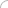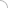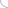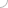Logical Reasoning## Online logical reasoning test

1. Find out the number that does not fit into the given series.

54, 48, 41, 34, 24

a.) 54
b.) 48
c.) 41
d.) 34

2. Find out the number that does not fit into the given series.

1, 3, 9, 27, 82

a.) 82
b.) 27
c.) 9
d.) 3

3. Complete the following series.

0, 7, 26, 63, 124, ……..

a.) 224
b.) 115
c.) 215
d.) 214

4. Complete the following series.

48, 43, 42, 37, 36, 31, 30, ……….

a.) 29
b.) 24
c.) 25
d.) 28

5. Complete the following series.

876, 765, 654, 543, …….

a.) 430
b.) 431
c.) 432
d.) None of these.

6. Complete the following series.

1, 3, 7, 15, 31. __, __

a.) 62, 127
b.) 63, 126
c.) 63, 127
d.) 62, 126

7. Complete the following series.

3, 4, 6, 9, 13, __, __

a.) 17, 25
b.) 17, 26
c.) 18, 24
d.) 18, 25

8. Find out the missing figure.

If 20 X 2 = 20                25 X 4 = 50                   then 30 X 8 = ?

a.) 100
b.) 110
c.) 120
d.) None of these.

9. Find out the missing figure.

If 8 -9 = 503            6 – 5 = 211                 then 7 – 4 = ?

a.) 337
b.) 338
c.) 339
d.) 340

10. Put + or – between the numbers to obtain the given result.

7    3     12    13    9 = 14

a.) + - + -
b.) + + + -
c.) - - + +
d.) - + - +

ANSWER : - - + +

11. In a two digit number, its digits are reversed and this new number is then added to the original number. The addition is always divisible by which of the following?

a.) 3
b.) 7
c.) 9
d.) 11

12. Which of the following is a perfect square?

a.) 19210
b.) 23435
c.) 28561
d.) 17466

13. A bus is hired at the rate of Rs. 320 per day for a picnic. Since some people did not turn up, the average contribution was Rs. 48 more per person per day than before. How many people were expected, and how many turned up?

a.) 8, 5
b.) 5, 2
c.) 10, 4
d.) 5, 4

14. If a coherent word can be formed out of the letters: USMOE, which is its fourth letter?

a.) E
b.) M
c.) U
d.) S

15. The letters A, B, C, D, E each stand for one of 1, 2, 3, 4 and 5, but not necessarily in that order. A is odd, B is neither 4 nor 5, C is 1, D is either 4 or 5 and E is none of 2, 3, 4. The correct order of the digits (according to the correct order of the English alphabet) is –

a.) 32145
b.) 52341
c.) 11245
d.) None of these

16. Which pair of numbers is different from the rest?

a.) (5, 15)
b.) (6, 24)
c.) (7, 49)
d.) (9, 63)

17. A box contains 6 red beads and 5 blue beads. What is the smallest number of beads that must be picked at a time, without looking, to be sure of getting two of the same colour?

a.) 3
b.) 7
c.) 2
d.) 11

18. In a certain language EGG is coded as 577, what should be the code for CAB?

a.) 312
b.) 213
c.) 123
d.) 321
e.) 314

19. In a certain language SEE is coded as 11, what will be the value for PLEA?

a.) 6
b.) 7
c.) 8
d.) 9
e.) 12

20. In certain language RUN is coded as 2, what will be the code for SUN?

a.) 5
b.) 6
c.) 1
d.) 3
e.) 7

21. In a certain language CAN coded as 414, what will be the code for FAR?

a.) 618
b.) 817
c.) 178
d.) 871
e.) 718Aptitude questions